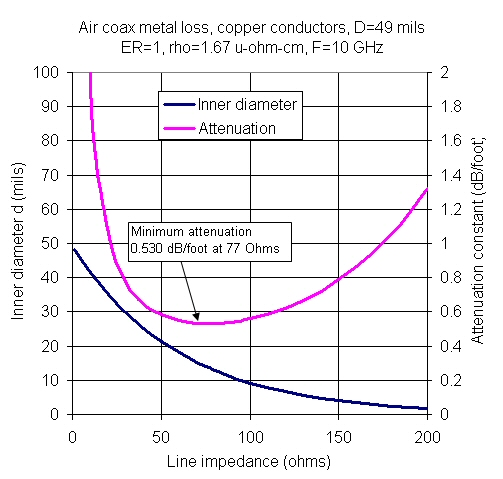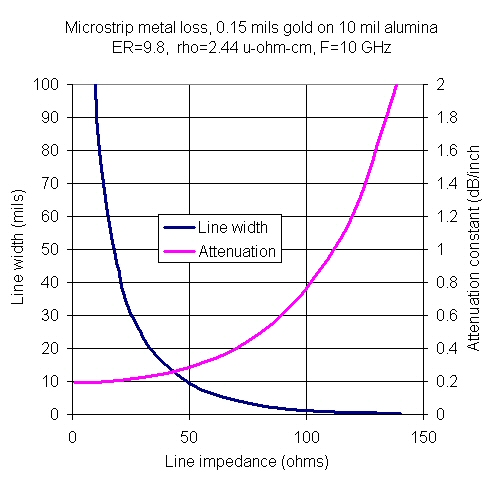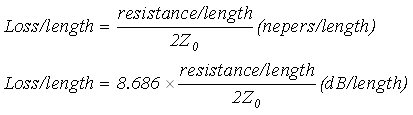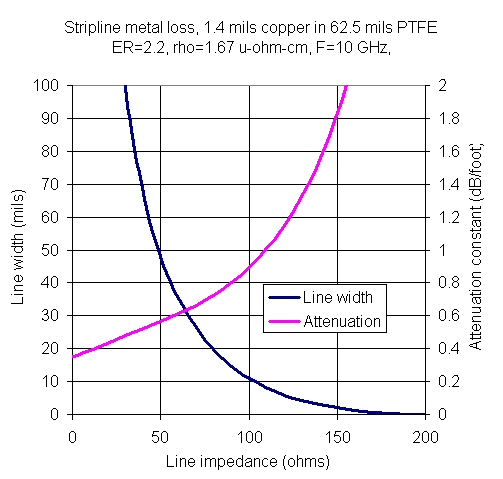# Minimizing Attenuation

Click here to go to our main page on transmission line loss

New for October 2007! He's information on minimizing loss of transmission lines, which is expressed as the attenuation factor in units of dB/length or Nepers/length. Of course, once you deviate from 50 ohms, you'll need to add transformers to get back to 50 ohms because it is the widely accepted standard for everything. OK, 75 ohms is also a standard, but it is not used as much as 50 ohms.

### Coax

Coax transmission lines exhibit a well-known minimum in attenuation versus line impedance. At zero ohms the loss/length is infinity, the same is true at infinity ohms.

Air coax has its minimum attenuation at 77 ohms. It is believed that this phenomenon is what caused the 75 ohm impedance standard for cables. The plot below is for air-coax, outer conductor D=0.049 inches, copper conductors, at a frequency of 10 GHz. Minimum loss is a little more than 0.5 dB for a foot of this cable (which you can't buy anywhere, no one makes air coax that small!)Remember, the minimum attenuation feature is also a function of the coax dielectric constant. For PTFE-filled cables, the minimum attenuation is nearly at 50 ohms (52 ohms by our calculation, which you can verify when you download our coax calculator Excel file!).

So, what's the story with microstrip and stripline? Is there a sweet spot on the impedance/attenuation function? We'll spoil the ending, the answer is "no!"

### Microstrip

The equations for microstrip are quite complicated, we'll avoid them and rely on Agilent's ADS software to look into this. We used a well-known microstrip media, 10 mil alumina circuit with gold conductors, at 10 GHz.The minimum attenuation is at Z0=0 ohms. Not only is this a useless impedance, but it seems strange that there is a finite loss under these conditions (shouldn't it be either zero or infinity?) What's going on here? Recall the loss per length calculation, resistance/length appears in the numerator, while characteristic impedance is in the denominator. Both are decreased as the line width increases, but not exactly proportionately.You could build up a closed form equation for microstrip loss/length as a function of line width, then take the limit as W goes to infinity and you'd come up with the non-zero value of attenuation at Z0=0. If anyone has the time, send us the math and we'll post it!

### Stripline

We used ADS to calculate the attenuation factor of a PTFE stripline board with copper conductor and popular geometry.

Stripline has the same result, loss is minimum as Z0=0. Although the curves would change if you played with parameters such as metal conductivity, dielectric constant, and geometry, trust us, the attenuation factor always decreases when impedance is reduced. This is a Microwaves101 Rule of Thumb!Author : Unknown Editor